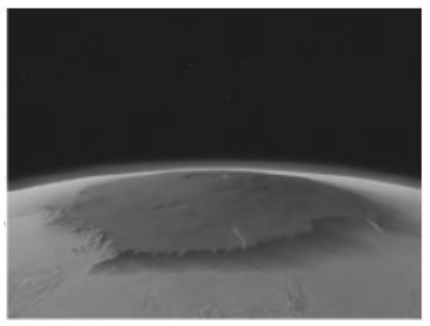Chapter 10.5, Problem 77EFinite Mathematics and Applied Cal...

7th Edition
Stefan Waner + 1 other
ISBN: 9781337274203

Solutions

Chapter
SectionFinite Mathematics and Applied Cal...

7th Edition
Stefan Waner + 1 other
ISBN: 9781337274203
Textbook Problem

Temperatures on Mars The air temperature one chilly spring morning at your time share condominium at the base of Olympus Mons, t hours after 6:00 am, was given by the function f ( t ) = − 5 t 2 + 50 − 80 degreesFahrenheit ( 0 ≤ t ≤ 4 ) .58 What was the temperature at 7:00 am, and how fast was it rising? (Use the method of Example 1(a)To determine

To calculate: The temperature at 7:00 am and the rate of rise in temperature at the base of Olympus Mons, when the air temperature at the base of Olympus Mons t hours after 6:00 am is provided as a function of time f(t)=5t2+50t80 degree Fahrenheit where (0t4).Explanation

Given Information:

The provided function is f(t)=5t2+50t80 where (0t4).

The image of Olympus Mons is given below:

Formula used:

The quick approximation of the derivative states that:

When f is differential at a, the approximate value of f(a) can be calculated as,

f(a)f(a+h)f(a)h

Where the value of h=0.0001.

Calculation:

Consider the function, f(t)=5t2+50t80.

The function f(t)=5t2+50t80 correspond to t=1 that is one hour after 6:00 am.

Estimate the value of instantaneous rate of change at t=1

f'(1)=f(1+h)f(1)h

Compute f(1+h) by substitute the value t=1+h in the function f(t)=5t2+50t80:

f(1+h)=5(1+h)2+50(1+h)80

Compute f(1) by substituting the value t=1 in the function f(t)=5t2+50t80:

f(1)=5(1)2+50(1)80=5+5080=4580=35

Substitute the values in the formula:

f(1)=[5(1+h)2+50(1+h)80](35)h=5(1+h)2+50(1+h)80+35h=5(1+h)2+50(1+h)45h

Compute the value of f(1) for different values of h=1, 0.1, 0.01, 0.001, 0.0001:

When h=1

f(1)=5(1+1)2+50(1+1)451=5(4)+50(2)45=20+10045=35

When h=0.1

f(1)=5(1+0.1)2+50(1+0.1)450.1=5(1.21)+50(1.1)450.1=6.05+55450.1=39.5

When h=0.01

f(1)=5(1+0.01)2+50(1+0.01)450.01=5(1.0201)+50(1.01)450.01=5.1005+50.5450.01=39.95

When h=0.001

f(1)=5(1+0.001)2+50(1+0.001)450.001=5(1.002001)+50(1.001)450.001=5.010005+50

Still sussing out bartleby?

Check out a sample textbook solution.

See a sample solution

The Solution to Your Study Problems

Bartleby provides explanations to thousands of textbook problems written by our experts, many with advanced degrees!

Get Started

In Exercises 63-68, rationalize the denominator. 66. 5x23x

Applied Calculus for the Managerial, Life, and Social Sciences: A Brief Approach

In Problems 17-20, use Excel or some other technology. 19. Minimize subject to

Mathematical Applications for the Management, Life, and Social Sciences

Evaluate the integral. 22. costdt

Single Variable Calculus: Early Transcendentals